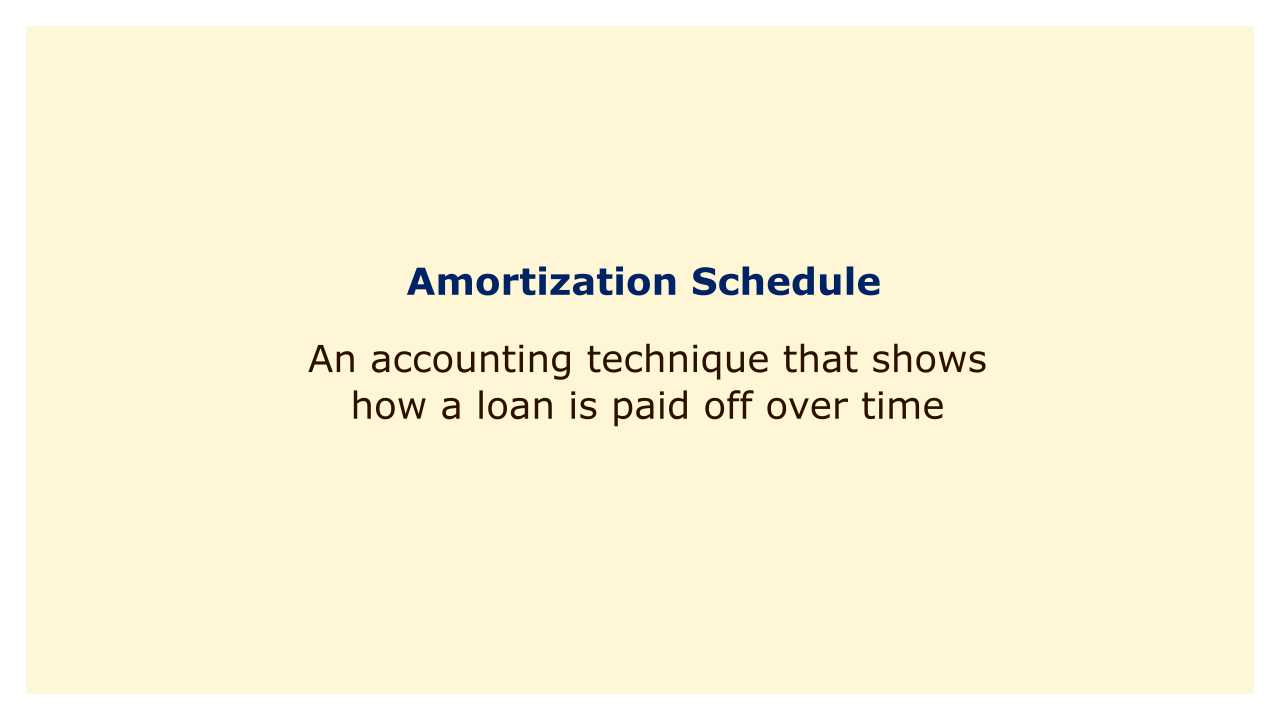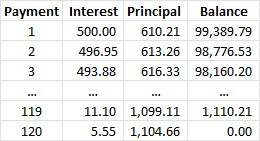# Amortization ScheduleImage: Moneybestpal.com

### The amortization schedule is an accounting method that illustrates the gradual repayment of a loan. The sum after each payment, the interest and principal components of each payment, and the monthly payments are all listed.

You can plan your budget accordingly by using an amortization schedule to better understand how much interest you will pay over the course of the loan, how quickly your debt will be paid off, and how much you will spend in total. You need to know the loan amount, the interest rate, the loan duration, and the frequency of payments in order to generate an amortization schedule. To create an amortization schedule, you can use an internet calculator, a spreadsheet, or a formula to manually calculate each payment.

The formula for calculating the monthly payment of a fixed-rate loan is:

P = rA / (1 - (1 + r)^-n)

where:
• P is the monthly payment
• r is the monthly interest rate (annual interest rate divided by 12)
• A is the loan amount
• n is the number of payments (loan term in months)

For example, if you borrow \$100,000 at 6% annual interest for 10 years, your monthly payment will be:

P = 0.06/12 x 100,000 / (1 - (1 + 0.06/12)^-120)

P = 1,110.21

To calculate the interest and principal portions of each payment, you can use these formulas:

I = rB

C = P - I

where:
• I is the interest payment
• r is the monthly interest rate
• B is the outstanding balance before the payment
• C is the principal payment
• P is the monthly payment

For example, for the first payment of the \$100,000 loan, the interest and principal portions will be:

I = 0.06/12 x 100,000

I = 500

C = 1,110.21 - 500

C = 610.21

To calculate the remaining balance after each payment, you can use this formula:

B = B - C

where:
• B is the outstanding balance before and after the payment
• C is the principal payment

For example, after the first payment of the \$100,000 loan, the remaining balance will be:

B = 100,000 - 610.21

B = 99,389.79

You can repeat these steps for each subsequent payment until the loan is fully paid off. The amortization schedule will look something like this:Image: Moneybestpal.com

You can see that as you pay more each month, the principal amount grows while the interest portion shrinks. In other words, you are accruing more debt while paying less interest.

You can compare several loan possibilities using an amortization schedule, create a budget, and monitor your debt repayment progress.
Tags